• 基于51单片机的交通灯
2021-06-25 16:09:00

按键说明：
从上到下，繁忙键，正常键，特殊键。单独一个按键为单片机复位按键。
操作说明：
打开仿真文件开始仿真，程序开始数码管倒计时，
1，东西方向为红灯，此道车辆禁止通行，东西道行人可通过；南北道为绿灯，此道车辆通过，行人禁止通行,时间为40秒。
2，黄灯闪烁5秒，警示车辆和行人红、绿灯的状态即将切换。
3，东西方向左拐，时间为20S
4，东西方向为绿灯，此道车辆通行；南北方向为红灯，南北道车辆禁止通过，行人通行。时间为40秒。
5，南北方向左拐，时间为20S
6，黄灯闪烁5秒，警示车辆和行人红、绿灯的状态即将切换。
没有按键按下情况下会一直这样循环运行下去。
繁忙键：直行时间改为45S，左转为15S
正常键：直行通行时间为40S，左转为20S
特殊键：这个按键按下侯数码管不显示数字，并且东西南北红灯一直亮。代表紧急模式。再次按下此键交通灯正常运行。

更多相关内容
• 本系统采用单片机AT80C51为中心器件来模拟十字路口交通灯的各种状态显示以及倒计时时间，系统实用性强、操作简单、扩展性强。 附件为程序C代码
• 基于51单片机交通灯课设(内含程序和实物图).docx基于51单片机交通灯课设(内含程序和实物图).docx基于51单片机交通灯课设(内含程序和实物图).docx基于51单片机交通灯课设(内含程序和实物图).docx基于51单片机交通灯...
• 基于51单片机交通灯课设(内含程序和实物图).pdf基于51单片机交通灯课设(内含程序和实物图).pdf基于51单片机交通灯课设(内含程序和实物图).pdf基于51单片机交通灯课设(内含程序和实物图).pdf基于51单片机交通灯课设...
• 本设计包括两个按键，单片机复位按键,特殊键。 程序运行后开始数码管倒计时，没有按键按下程序循环运行。 1，东西方向为绿灯，行人和车辆东西方向可以同行东西绿灯时间长20s。 2，东西方向黄灯闪烁5秒，南北方向还是...
• 基于C51单片机交通灯设计（软件源程序+硬件原理图+proteus仿真+设计文档资料） 本设计以单片机为核心，以LED数码管作为倒计时指示，根据设计的要求我们考虑了各功能模块的几种设计方案，以求最佳方案，实现实时显示...
• 1、资源内容：源程序、原理图、仿真 2、学习目标：快速完成相关题目设计； 3、应用场景：课程设计、diy、毕业、参赛； 4、特点：word版，直接可以编辑使用； 5、使用人群：设计参赛人员，学生，教师等。...
• 单独一个按键为单片机复位按键。 操作说明： 打开仿真文件开始仿真，程序开始数码管倒计时， 1，东西方向为红灯，此道车辆禁止通行，东西道行人可通过；南北道为绿灯，此道车辆通过，行人禁止通行,时间为40秒。 2，...
• 基于51单片机的十字路口交通灯控制系统设计(含源码及仿真图) (1)东西、南北方向各设有一个绿、黄、红指示灯，两个显示数码管。 (2)两个方向交替允许通行，基本放行时间为25s，另外有黄灯闪烁5s。 (3)控制人员可以...
• 1.显示顺序循环：东西方向一致；绿、黄、红，南北方向一致；红、绿、黄。 2.在仿真里放置四个七段数码管，以倒计时的方式显示通行或禁止通行时间，其中东西方向红灯的显示时间是20s，与此同时，南北方向绿灯亮的时间...
• 基于C51单片机交通灯课设打包，内含源代码、professional画图等
• 交通灯程序设计 交通灯protues仿真程序基于51单片机交通灯仿真
• 废话少说直接上图 ...提取码：azxy #include"reg52.h" //头文件 ... //红色 勇敢牛牛路前面3个 sbit P12=P1^2; //绿色 sbit P13=P1^3; //黄色 sbit P14=P1^4; //红色 不怕困难路右面3个 sbit P15=P1^

废话少说直接上图https://pan.baidu.com/s/15BvnC4jLvHe2zWJLPi4qlg 提取码：azxy

#include"reg52.h"  //头文件

typedef unsigned char u8;
typedef unsigned int u16;

sbit P10=P1^0; //黄色灯
sbit P11=P1^1; //红色灯	   勇敢牛牛路前面3个灯
sbit P12=P1^2; //绿色灯

sbit P13=P1^3; //黄色灯
sbit P14=P1^4; //红色灯	   不怕困难路右面3个灯
sbit P15=P1^5; //绿色灯

sbit P16=P1^6; //黄色灯
sbit P17=P1^7; //红色灯	   勇敢牛牛路后面3个灯
sbit P30=P3^0; //绿色灯

sbit P31=P3^1; //黄色灯
sbit P32=P3^2; //红色灯	   不怕困难路左面3个灯
sbit P33=P3^3; //绿色灯

sbit P34=P3^4; //勇敢牛牛路直行按钮
sbit P35=P3^5; //不怕困难路直行按钮

u8 smg_display;
u8 code wala={0x01,0x02,0x04,0x08,0x10,0x20,0x40,0x80}; //数码管位选
u8 code duan={0xc0,0xf9,0xa4,0xb0,0x99,0x92,0x82,0xf8,0x80,0x90}; //数码管 0~9
u8 s=0;
/*************************************************
*********************延迟函数*********************
*************************************************/
void delay(u16 i)
{
while(i--);
}
/*************************************************
********************定时器中断********************
*************************************************/
void Timer0Init(void)		//5毫秒@11.0592MHz
{
TMOD &= 0xF0;		//设置定时器模式
TL0 = 0x00;		//设置定时初值
TH0 = 0x28;		//设置定时初值
TF0 = 0;		//清除TF0标志
TR0 = 1;		//定时器0开始计时
ET0=1;
EA=1;
}
/*************************************************
******************数码管显示函数******************
*************************************************/
void smg_buff()
{
static u8 num=0;
while(num<8)
{
P2=~wala[num];
P0=smg_display[num];
num++;
delay(200);
}
if(num==8) num=0;
}
/*************************************************
******************交通灯显示函数******************
*************************************************/
void smg_led()
{
if(s==70)
{
s=0;
}
if(s<30)
{
smg_display=~duan[(30-s)/10];	//勇敢牛牛路前面时间
smg_display=~duan[(30-s)%10];

smg_display=~duan[(35-s)/10];	//不怕困难路右面时间
smg_display=~duan[(35-s)%10];

smg_display=~duan[(30-s)/10];	//勇敢牛牛路后面时间
smg_display=~duan[(30-s)%10];

smg_display=~duan[(35-s)/10];	//不怕困难路左面时间
smg_display=~duan[(35-s)%10];

P10=0;P11=0;P12=1;	//黄 红 绿	   勇敢牛牛路前面3个灯
P13=0;P14=1;P15=0;	//黄 红 绿	   不怕困难路右面3个灯
P16=0;P17=0;P30=1;	//黄 红 绿	   勇敢牛牛路后面3个灯
P31=0;P32=1;P33=0;	//黄 红 绿	   不怕困难路左面3个灯
}
else if(s<35)
{
smg_display=~duan[(35-s)/10];    //勇敢牛牛路前面时间
smg_display=~duan[(35-s)%10];

smg_display=~duan[(35-s)/10];	//不怕困难路右面时间
smg_display=~duan[(35-s)%10];

smg_display=~duan[(35-s)/10];	//勇敢牛牛路后面时间
smg_display=~duan[(35-s)%10];

smg_display=~duan[(35-s)/10];	//不怕困难路左面时间
smg_display=~duan[(35-s)%10];

P10=1;P11=0;P12=0;  //黄 红 绿	   勇敢牛牛路前面3个灯
P13=0;P14=1;P15=0;	//黄 红 绿	   不怕困难路右面3个灯
P16=1;P17=0;P30=0;	//黄 红 绿	   勇敢牛牛路后面3个灯
P31=0;P32=1;P33=0;	//黄 红 绿	   不怕困难路左面3个灯
}
else if(s<65)
{
smg_display=~duan[(70-s)/10];	//勇敢牛牛路前面时间
smg_display=~duan[(70-s)%10];

smg_display=~duan[(65-s)/10];	//不怕困难路右面时间
smg_display=~duan[(65-s)%10];

smg_display=~duan[(70-s)/10];	//勇敢牛牛路后面时间
smg_display=~duan[(70-s)%10];

smg_display=~duan[(65-s)/10];	//不怕困难路左面时间
smg_display=~duan[(65-s)%10];

P10=0;P11=1;P12=0;	//黄 红 绿	   勇敢牛牛路前面3个灯
P13=0;P14=0;P15=1;	//黄 红 绿	   不怕困难路右面3个灯
P16=0;P17=1;P30=0;	//黄 红 绿	   勇敢牛牛路后面3个灯
P31=0;P32=0;P33=1;	//黄 红 绿	   不怕困难路左面3个灯
}
else
{
smg_display=~duan[(70-s)/10];	//勇敢牛牛路前面时间
smg_display=~duan[(70-s)%10];

smg_display=~duan[(70-s)/10];	//不怕困难路右面时间
smg_display=~duan[(70-s)%10];

smg_display=~duan[(70-s)/10];	//勇敢牛牛路后面时间
smg_display=~duan[(70-s)%10];

smg_display=~duan[(70-s)/10];	//不怕困难路左面时间
smg_display=~duan[(70-s)%10];

P10=0;P11=1;P12=0;	//黄 红 绿	   勇敢牛牛路前面3个灯
P13=1;P14=0;P15=0;	//黄 红 绿	   不怕困难路右面3个灯
P16=0;P17=1;P30=0;	//黄 红 绿	   勇敢牛牛路后面3个灯
P31=1;P32=0;P33=0;	//黄 红 绿	   不怕困难路左面3个灯
}

}
/*************************************************
*********************直行函数*********************
*************************************************/
void green()
{
if(P34==0)
{
TR0 = 0;
smg_display=0x00;	//勇敢牛牛路前面时间
smg_display=0x00;

smg_display=0x00;	//不怕困难路右面时间
smg_display=0x00;

smg_display=0x00;	//勇敢牛牛路后面时间
smg_display=0x00;

smg_display=0x00;	//不怕困难路左面时间
smg_display=0x00;

P10=0;P11=0;P12=1;	 //黄 红 绿	   勇敢牛牛路前面3个灯
P13=0;P14=0;P15=0;	 //黄 红 绿	   不怕困难路右面3个灯
P16=0;P17=0;P30=1;	 //黄 红 绿	   勇敢牛牛路后面3个灯
P31=0;P32=0;P33=0;	 //黄 红 绿	   不怕困难路左面3个灯
}
else
{
TR0 = 1;
}
if(P35==0)
{
TR0 = 0;
smg_display=0x00;	//勇敢牛牛路前面时间
smg_display=0x00;

smg_display=0x00;	//不怕困难路右面时间
smg_display=0x00;

smg_display=0x00;	//勇敢牛牛路后面时间
smg_display=0x00;

smg_display=0x00;	//不怕困难路左面时间
smg_display=0x00;

P10=0;P11=0;P12=0;	 //黄 红 绿	   勇敢牛牛路前面3个灯
P13=0;P14=0;P15=1;	 //黄 红 绿	   不怕困难路右面3个灯
P16=0;P17=0;P30=0;	 //黄 红 绿	   勇敢牛牛路后面3个灯
P31=0;P32=0;P33=1;	 //黄 红 绿	   不怕困难路左面3个灯
}
else
{
TR0 = 1;
}
}
/*************************************************
*********************主函数*********************
*************************************************/
void main()
{
P1=0x00;P3=0x00;
P34=1;P35=1;
Timer0Init();
while(1)
{
smg_led();
green();
smg_buff();
}
}
/*************************************************
********************中断服务函数*******************
*************************************************/
void timer0() interrupt 1
{
static u8 i=0;
i++;
if(i==200)	   //定时1S
{
i=0;
s++;
}

}

展开全文• 本文档为基于51单片机交通灯控制系统设计报告,本人原创,需要的同学可以下载参考,相互交流学习,由于作者水平有限,若有不足之处欢迎指正。
• 交通灯程序设计+交通灯protues仿真程序基于51单片机交通灯仿真
• Deli软件基于语言，利用51单片机开发板外接电路实现模拟十字路口交通灯状况，TL-1到TL-5功能逐步完善。TL-5能实现自己设置各个路口的亮灯时间。具体外接电路设计图也在压缩包中可配套使用。
• 采用STC89C51芯片作为中心期间来设计交通灯来控制电路，结合七段共阴极数码管显示时间的模块、交通灯显示模块、按键电路、IO口扩展电路等组成，通过程序设计和软件仿真来实现红、黄、绿、蓝燃亮时间以及双位数码管...
基于51单片机交通灯程序设计与仿真
摘要

交通信号灯是城市交通安全、有序、快速运行的重要保障。本文提出一种基于单片机的交通信号灯控制系统的设计方案。该系统模拟现实中十字路口的通行指示，倒计时、紧急车辆通行、强制东西/南北通行、夜间模式灯、转向等功能。并结合交通灯，设计了人行道灯。采用STC89C51芯片作为中心期间来设计交通灯来控制电路，结合七段共阴极数码管显示时间的模块、交通灯显示模块、按键电路、IO口扩展电路等组成，通过程序设计和软件仿真来实现红、黄、绿、蓝燃亮时间以及双位数码管显示倒计时。

关键字:交通信号灯、人行道灯、STC89C51单片机、紧急通行、转向功能

51单片机交通灯Proteus仿真

## 一、工程介绍

### 1.1、实现要求

1. 控制交通灯实现车辆东西通行后，南北通行功能
2. 控制转向灯实现交通灯东西通行后左转，南北通行后左转功能
3. 控制人行道灯实现交通灯东西通行的同时，同时南北人行道开启，同理南北通行亦如此
4. 可以控制按钮实现东西时间的调整、夜间模式、紧急模式、强制东西/南北模式、查看时间

## 二、仿真电路设计

### 2.2、电路设计

#### 2.2.1、最小系统的实现

关于51单片机最小系统可以查看我以前博客，2.2.1、最小系统实现参考链接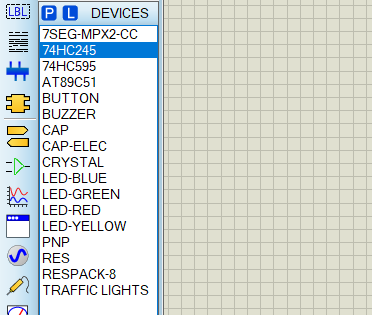从Proteus中选取元件有：74HC595芯片、74HC245芯片、AT89C51单片机、BUTTON开关、BUZZER蜂鸣器、CAP电容、CAP-ELEC电解电容、CRYSTAL晶振、LED-BLUE蓝色LED、LED-GREEN绿色LED、LED－RED红色LED、LED－YELLOW黄色LED、PNP三极管、RES电阻、RESPACK-8排阻、TRAFFICLIGHTS交通灯、7SEG-MPX2-CC两位八段共阴极数码管

最小系统如下：#### 2.2.2、数码管的使用

数码管按段数可分为七段数码管和八段数码管，八段数码管比七段数码管多一个发光二极管单元（多一个小数点显示）；按能显示多少个“8”可分为1位、2位、3位、4位、5位、6位、7位等数码管。按发光二极管单元连接方式可分为共阳极数码管和共阴极数码管。共阳数码管是指将所有发光二极管的阳极接到一起形成公共阳极(COM)的数码管，共阳数码管在应用时应将公共极COM接到+5V，当某一字段发光二极管的阴极为低电平时，相应字段就点亮，当某一字段的阴极为高电平时，相应字段就不亮。共阴数码管是指将所有发光二极管的阴极接到一起形成公共阴极(COM)的数码管，共阴数码管在应用时应将公共极COM接到地线GND上，当某一字段发光二极管的阳极为高电平时，相应字段就点亮，当某一字段的阳极为低电平时，相应字段就不亮。

一位数码管内部原图如图所示：在实际电路中数码管是要加驱动芯片的，因为单靠51单片机管脚输出电流是不够的，这里通过添加74HC245驱动芯片驱动各个数码管，用51单片机P0口传送数据给数码管，一定要加上拉电阻，并通过八同相三态总线收发器74HC245直接连接数码管的八个LED。

74HC245芯片内部结构如下: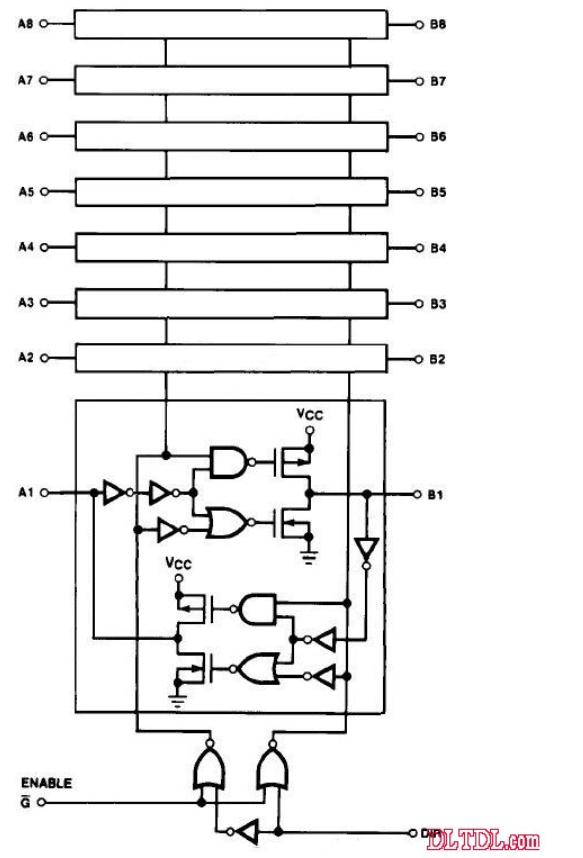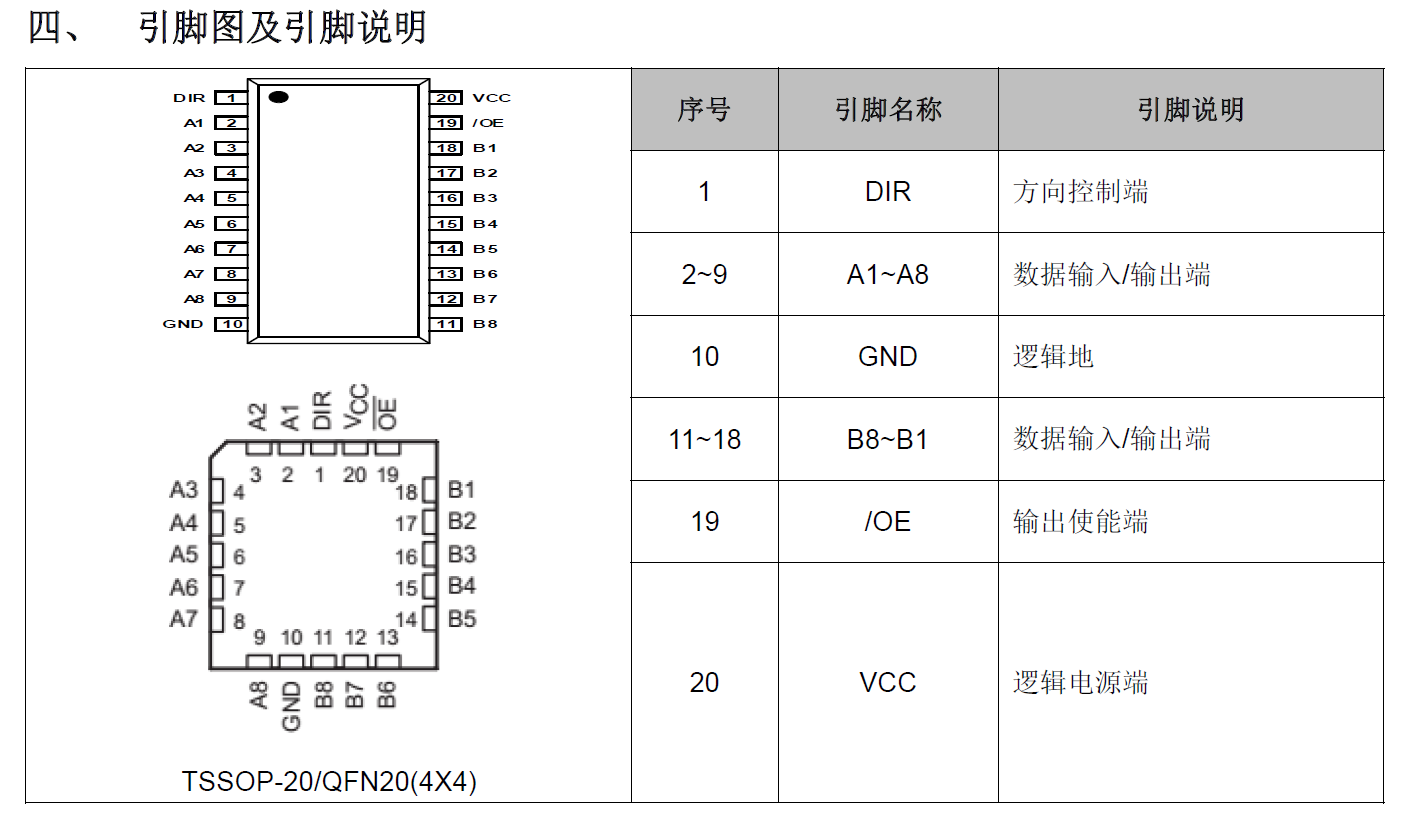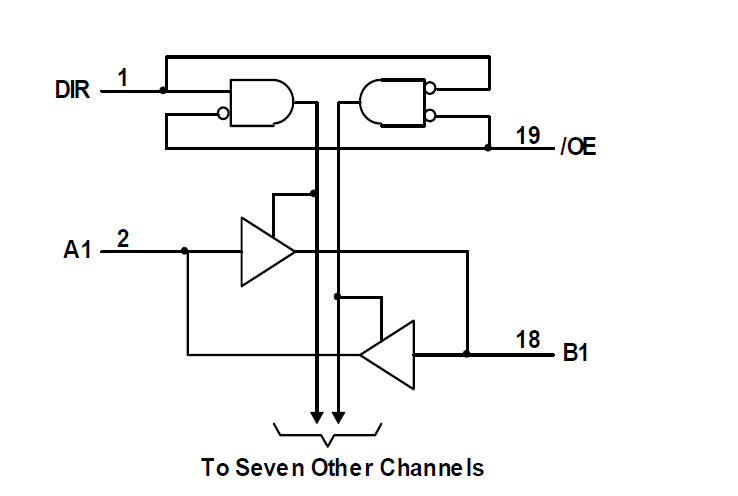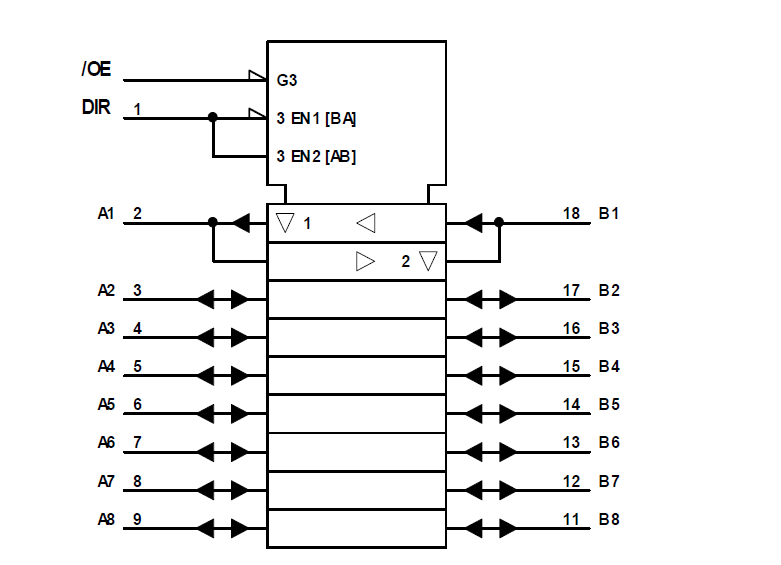由于单片机或CPU的数据地址控制总线端口都有一定的负载能力，如果负载超过其负载能力，一般应加驱动器。主要应用于大屏显示,以及其它的消费类电子产品中增加驱动。

仿真电路图如下：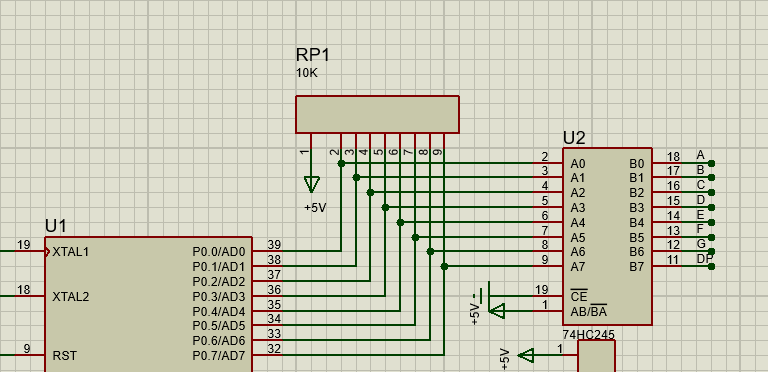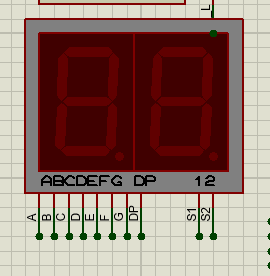#### 2.2.3、交通灯、人行灯及转向灯

通过Proteus绘制路线以及人行道，可以画出如下电路图：#### 2.2.4、74HC595芯片的使用

74HC595是串口转并口芯片,可输出三种状态:高电平、低电平和高组态。一片74HC595芯片可实现3根口线扩展为8根口线.也可采用多片74HC595进行级联的方式扩展输出口线。控制可以采取如下控制：仿真电路图如下:#### 2.2.6、蜂鸣器电路的设计当IO输出低电平时，三极管导通，蜂鸣器发出声音。

#### 2.2.7、按键电路的设计### 2.3、总体仿真电路图## 三、程序设计

### 3.2、定时器的使用

89C51单片机内部有两个16位的定时/计数器，即定时器T0和定时器T1，这次仿真是要控制数码管每隔1S变化一次，所以应把两个定时器配置50MS的中断，响应10次即完成0.5S，响应20次完成1S。由于51单片机1个机器周期=12个时钟周期，系统时钟频率为12MHZ，则一个机器周期为1US，$Sysclk = \frac{12MHZ}{12}=1MHZ$。下面是涉及定时器的相关寄存器：工作模式寄存器TMOD是用于控制定时器0/1的工作模式，通过对TMOD进行赋值，则可以改变定时器的工作模式具体各位的定义如下：定时器T0/T1有四种工作模式：模式0（13位定时器/计数器），模式1（16位定时器/计数器模式），模式2（8位自动重重装载模式），模式3（两个8位定时器/计数器）。T1除模式3外，其他工作模式与定时器/计数器0相同，T1载模式3无效，停止计数。

定时器的控制寄存器TCON为定时器/计数器T0、T1的控制寄存器，同时也锁存T0、T1溢出中断源和外部请求中断源等，格式如下：配置T0和T1定时器程序如下:

void TIMER_init(void)     // 定时器初始化配置
{
TMOD=0X11;            // 设置T0和T1为模式1（16位定时器）
TH1=0X3C;		     // 定时器1置初值 0.05S
TL1=0XB0;
TH0=0X3C;		     // 定时器0置初值 0.05S
TL0=0XB0;
EA=1;				 // 开总中断
ET0=1;				 // 定时器0中断开启
ET1=1;				 // 定时器1中断开启
TR0=1;				 // 启动定时0
TR1=0;				 // 关闭定时1
}


定时器T0实现交通灯和转向灯程序流程：

黄灯和转向灯闪烁程序如下:

void Yellow_Flicker(void)
{
if (countt0 == 10)						                    // 加到10也就是半秒
{
if ((sec_nb <= 5) && (dx_nb == 0) && (shanruo == 1))  	// 东西黄灯闪
{
Green_dx = 0;
Yellow_dx = 0;
if (DX_LL == 1)
SendTo595(0XF7);
Buzz = 0;						                        // 蜂鸣器关
}
if ((sec_dx <= 5) && (dx_nb == 1) && (shanruo == 1)) // 南北黄灯闪
{
Green_nb = 0;
Yellow_nb = 0;
if (NB_LL == 1)
SendTo595(0XFB);
Buzz = 0;					                          // 蜂鸣器关
}
}
if (countt0 == 20)	      // 定时器中断次数=20时（即1秒时）
{
if ((sec_nb <= 5) && (dx_nb == 0) && (shanruo == 1))  	// 打开交通灯
{
Green_dx = 0;
Buzz = 1;						                        // 蜂鸣器关
if (DX_LL == 1)
SendTo595(DX_L);
else
Yellow_dx = 1;
}
if ((sec_dx <= 5) && (dx_nb == 1) && (shanruo == 1))  // 南北黄灯闪
{
Green_nb = 0;
Buzz = 1;						                       // 蜂鸣器关
if (NB_LL == 1)
SendTo595(NB_L);
else
Yellow_nb = 1;
}
}
}


定时器0中断程序如下:

void time0(void) interrupt 1 using 1  	 // 定时中断子程序
{
TH0=0X3C;							                 // 重赋初值
TL0=0XB0;							                 // 12m晶振50ms//重赋初值
TR0=1;								                 // 重新启动定时器
countt0++;							               // 软件计数加1
Yellow_Flicker();

if(countt0==20)	      // 定时器中断次数=20时（即1秒时）
{	countt0=0;					// 清零计数器
sec_dx--;						// 东西时间减1
sec_nb--;						// 南北时间减1

if(sec_dx==0&&sec_nb==5) 		//当东西倒计时到0时，重置5秒，用于黄灯闪烁时间
{
sec_dx=5;
shanruo=1;
}
if(sec_nb==0&&sec_dx==5)		//当南北倒计时到0时，重置5秒，用于黄灯闪烁时间
{
sec_nb=5;
shanruo=1;
}
if(dx_nb==0&&sec_nb==0&&DX_LL==0)			//当东西通行时间完毕，开始东西左转
{
sec_nb = 10;
sec_dx = 10;
SendTo595(DX_L);
DX_LL=1;
Yellow_dx = 0;
}
if(dx_nb==0&&sec_nb==0&&DX_LL==1)			//当黄灯闪烁时间倒计时到0时，
{
Buzz=1;						//蜂鸣器开
P2 &=0x81;					//重置东西南背方向的红绿灯
Green_nb=1;
Red_dx=1;
dx_nb=!dx_nb;
shanruo=0;
if(num_che_nb>set_timenb/2)//如果此时南北通行的车辆数大于预设通行量
set_timenb=set_timenb+5;
if(num_che_nb==0)//如果南北方向无车辆通行,每次递减5秒
set_timenb=set_timenb-5;
if(set_timenb<=15)
set_timenb=15;
sec_nb=set_timenb;			//重赋南北方向的起始值
sec_dx=set_timenb+5;		//重赋东西方向的起始值
num_che_nb=0;//清零
SendTo595(NB_R);
DX_LL=0;
}
if(dx_nb==1&&sec_dx==0&&NB_LL==0)			//当南北通行时间完毕，开始南北左转
{
sec_nb = 10;
sec_dx = 10;
SendTo595(NB_L);
NB_LL=1;
Yellow_nb = 0;
}
if(dx_nb==1&&sec_dx==0&&NB_LL==1)			//当黄灯闪烁时间到
{
P2&=0X81;						//重置东西南北的红绿灯状态
Green_dx=1;					 //东西绿灯亮
Red_nb=1;					 //南北红灯亮
dx_nb=!dx_nb;				 //取反
shanruo=0;					//闪烁
if(num_che_dx>set_timedx/2)//如果此时南北通行的车辆数大于预设通行量
set_timedx=set_timedx+5;
if(num_che_dx==0)//如果东西方向无车辆通行,每次递减5秒
set_timedx=set_timedx-5;
if(set_timedx<=15)
set_timedx=15;
sec_dx=set_timedx;			//重赋东西方向的起始值
sec_nb=set_timedx+5;		//重赋南北方向的起始值
num_che_dx=0;//清零
SendTo595(DX_R);
NB_LL=0;
}

}
}


### 3.3、外部中断的使用

程序使用两个外部中断实现东西/南北强制转换，分别使用了外部中断0和外部中断1，且中断结构如下。通过结构图可以看出使用外部中断步骤如下：

1. 设置电平触发方式
2. 开启EX0/1
3. 开启总中断

初始化外部中断程序如下：

	IT0 = 1;//设置为下降沿触发
EX0 = 1;//使能外部中断
IT1 = 1;
EX1 = 1;


两个外部中断具体使用的功能：

//外部中断0
void int0(void) interrupt 0 using 1	   //只允许东西通行
{
TR0=0;								//关定时器0
TR1=0;								//关定时器1
P2=0x00;							//灭显示
Green_dx=1;							//东西方向置绿灯
Red_nb=1;							//南北方向为红灯
sec_dx=00;							//四个方向的时间都为00
sec_nb=00;
SendTo595(null);
}
//外部中断1
void int1(void) interrupt 2 using 1	  	 //只允许南北通行
{
TR0=0;								//关定时器0
TR1=0;							   //关定时器1
P2=0x00;							//灭显示
Green_nb=1;							//置南北方向为绿灯
Red_dx=1;							//东西方向为红灯
sec_nb=00;							//四个方向的时间都为00
sec_dx=00;
SendTo595(null);
}


### 3.4、控制595芯片

我们都知道通信从大的方面有两种：串行和并行。串行的最大优点是占用总线少，但是传输速率低；并行恰恰相反，占用总线多，传输速率高。市面上有很多这样的芯片，有串入并出的（通俗讲就是 一个一个进，最后一块出来），有并入串出的（相对前者而言）。具体用哪种类型要根据我们得实际情况。比如利用单片机显示数码管单纯的显示一个数码管如果仅仅是为了显示 那么动用单片机一个端口（如P0或P1/P2/P3）那没有什么，当然这里我说的数码管是8段的（如果利用BCD类型 16进制数码管那么只需四个即可）就拿51类型的单片机来说，总共32个I/O口，一般如果不是做太大的工程是完全够用的，但有些时候你会恨单片机怎么不多长几条“腿”，怎么省还是不够用。这个时候就需要用到并转串或者串转并芯片来进行IO口的扩展，74HC595就是一种串行转并行的芯片。参考链接

程序如下：

#define NB_L 0XFE
#define DX_L 0XFD
#define NB_G 0XF7
#define NB_R 0XF7
#define DX_G 5
#define DX_R 0XFB
#define null 0XF3
sbit DS = P2^0;
sbit SH_CP = P2^7;
sbit ST_CP = P1^4;
void SendTo595(uchar Data)//发送一个字节数据给595再并行输出
{
char i=0;
ST_CP = 0;
for(i;i<8;i++)
{
SH_CP = 0;
DS=0x80&Data;//&为按位运算符，即全1为1，有0为0，上式也就是 （1000 0000）&（1111 1111）=1000 0000，若高位为1则是1高位为0则这个式子为0
Data=_crol_(Data,1); //左移一位 将高位补给低位，如果二进制数为01010101 那么_crol_(1) 为10101010
SH_CP = 1;           //上升沿让串行输入时钟变成高电平 并延时一个时钟周期
_nop_();
}
/*位移寄存器完毕，转移到存储寄存器*/
ST_CP = 1;               //上升沿，存储寄存器变为高电平 延迟两个时钟周期
_nop_();
_nop_();
}


### 3.5、数码管程序设计

uchar code table={	//共阴极字型码
0x3f,  //--0
0x06,  //--1
0x5b,  //--2
0x4f,  //--3
0x66,  //--4
0x6d,  //--5
0x7d,  //--6
0x07,  //--7
0x7f,  //--8
0x6f,  //--9
0x00   //--NULL
};
void display(void) //显示子程序
{

if(xianshi_fx==0)//正常显示
{
buf=sec_nb/10; 		//第1位 东西显示秒十位
buf=sec_nb%10; 		//第2位 东西显示秒个位
buf=sec_dx/10; 		//第3位 南北显示秒十位
buf=sec_dx%10; 		//第4位 南北显示秒个位
}
if(xianshi_fx==1)//查看通行时间
{
buf=set_timenb/10; 		//第1位 东西通行秒十位
buf=set_timenb%10; 		//第2位 东西通行秒个位
buf=set_timedx/10; 		//第3位 南北通行秒十位
buf=set_timedx%10; 		//第4位 南北通行秒个位
}

if(xianshi_fx==2)//查看红外计数值
{
buf=num_che_nb/10; 		//第1位 东西红外计数值十位
buf=num_che_nb%10; 		//第2位 东西红外计数值个位
buf=num_che_dx/10; 		//第3位 南北红外计数值十位
buf=num_che_dx%10; 		//第4位 南北红外计数值个位
}
s1 = 1;s2 = 1;s3 = 1;s4 = 1;
P0=0x00;				 灭显示
s1 = 0;s2 = 1;s3 = 1;s4 = 1;
P0=table[buf];		//送东西时间十位的数码管编码
delay(1);				//延时
s1 = 1;s2 = 1;s3 = 1;s4 = 1;
P0=0x00;				//灭显示
s1 = 1;s2 = 0;s3 = 1;s4 = 1;
P0=table[buf];		 //送东西时间个位的数码管编码
delay(1);				 //延时
s1 = 1;s2 = 1;s3 = 1;s4 = 1;
P0=0x00;				//关显示
s1 = 1;s2 = 1;s3 = 0;s4 = 1;
P0=table[buf];		//送南北时间十位的数码管编码
delay(1);				//延时
s1 = 1;s2 = 1;s3 = 1;s4 = 1;
P0=0x00;				 //关显示
s1 = 1;s2 = 1;s3 = 1;s4 = 0;
P0=table[buf];		//送南北时间个位的数码管编码
delay(1);				//延时
}


## 五、结果分析与改进

1. 问题：仿真结果表明，当增加时间或者减小时间那么东西和南北的倒计时会同时增加
2. 解决：通过设置独立按键把东西/南北倒计时时间分开即可解决

## 参考资料：

展开全文• 摘要：AT89C51单片机交通灯控制系统是由AT89C51单片机、键盘电路、LED倒计时、交通灯显示等模块组成。系统除基本交通灯功能外，还具有通行时间手动设置、可倒计时显示、急车强行通过、交通特殊情况处理等相关功能...
• 基于51单片机的简单交通灯设计，里面包含C代码以及PCB原理图...